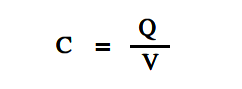# Capacitance

Capacitance is the ability to store an electrical charge. Capacitance is equal to the amount of charge that can be stored divided by the applied voltage, as shown in below Equation.where

C = capacitance (F)
Q = amount of charge (C)
V = voltage (V)

The unit of capacitance is the farad (F). A farad is the capacitance that will store one coulomb of charge when one volt is applied across the plates of the capacitor.

The dielectric constant (K) describes the ability of the dielectric to store electrical energy. Air is used as a reference and is given a dielectric constant of 1. Therefore, the dielectric constant is unitless. Some other dielectric materials are paper, teflon, bakelite, mica, and ceramic.

The capacitance of a capacitor depends on three things.

1. Area of conductor plates
2. Separation between the plates
3. Dielectric constant of insulation material

The below Equation illustrates the formula to find the capacitance of a capacitor with two parallel plates.where

C = capacitance
K = dielectric constant
A = area
d = distance between the plates
8.85 x 10-12 = constant of proportionality

Example 1:

Find the capacitance of a capacitor that stores 8 C of charge at 4 V.

C = Q/V

C = 8/4 = 2F

Example 2:

What is the charge taken on by a 5F capacitor at 2 volts?

Q = CV

Q = 5F x 2 V = 10 C

Example 3:

What is the capacitance if the area of a two plate mica capacitor is 0.0050 m2 and the separation between the plates is 0.04 m? The dielectric constant for mica is 7.C = 7 (0.005/0.04) 8.85 x 10-12

C = 7.74 x  10-12  F

C = 7.74 pF

Don't Miss Our Updates
Be the first to get exclusive content straight to your email.
We promise not to spam you. You can unsubscribe at any time.